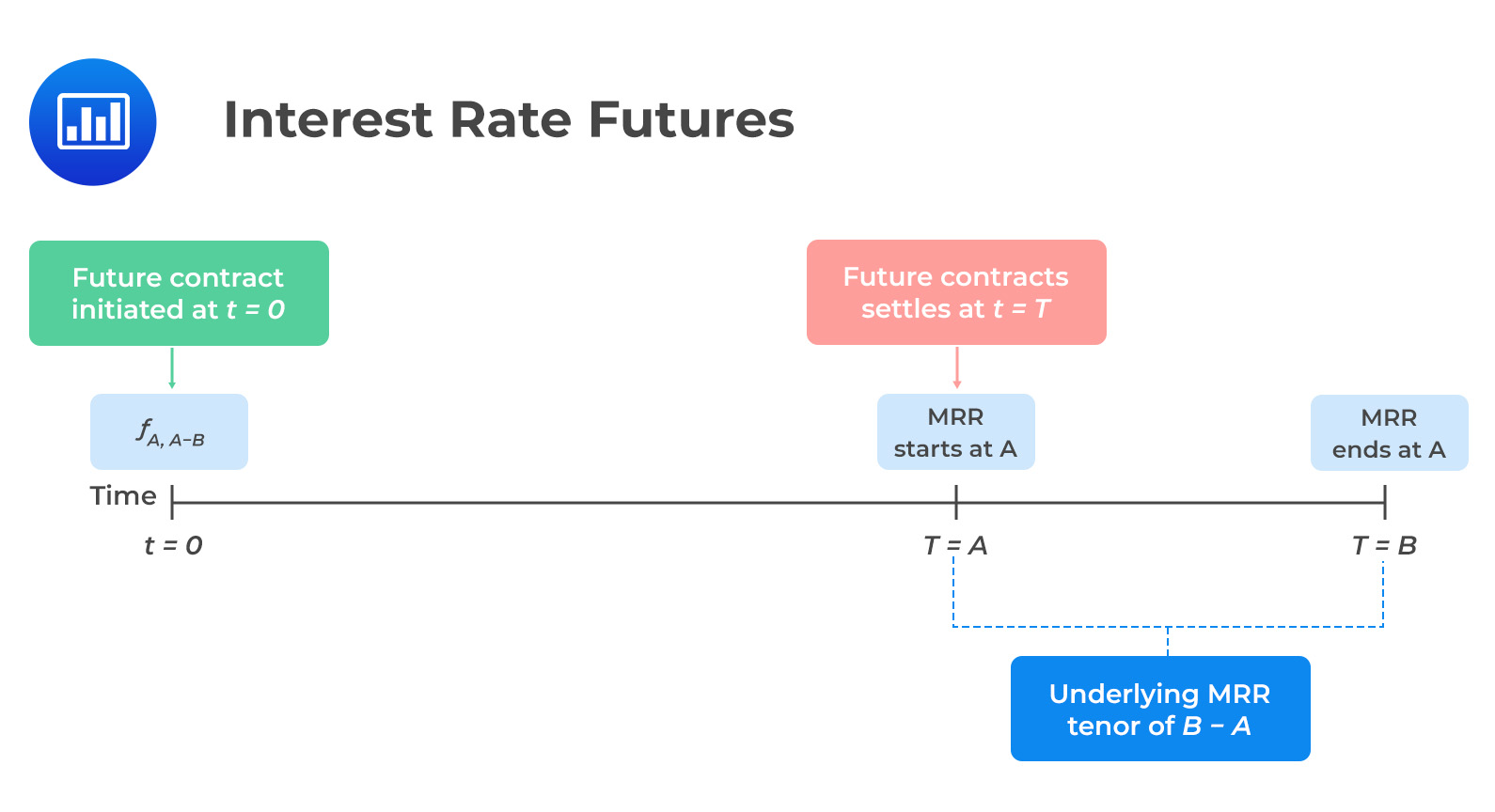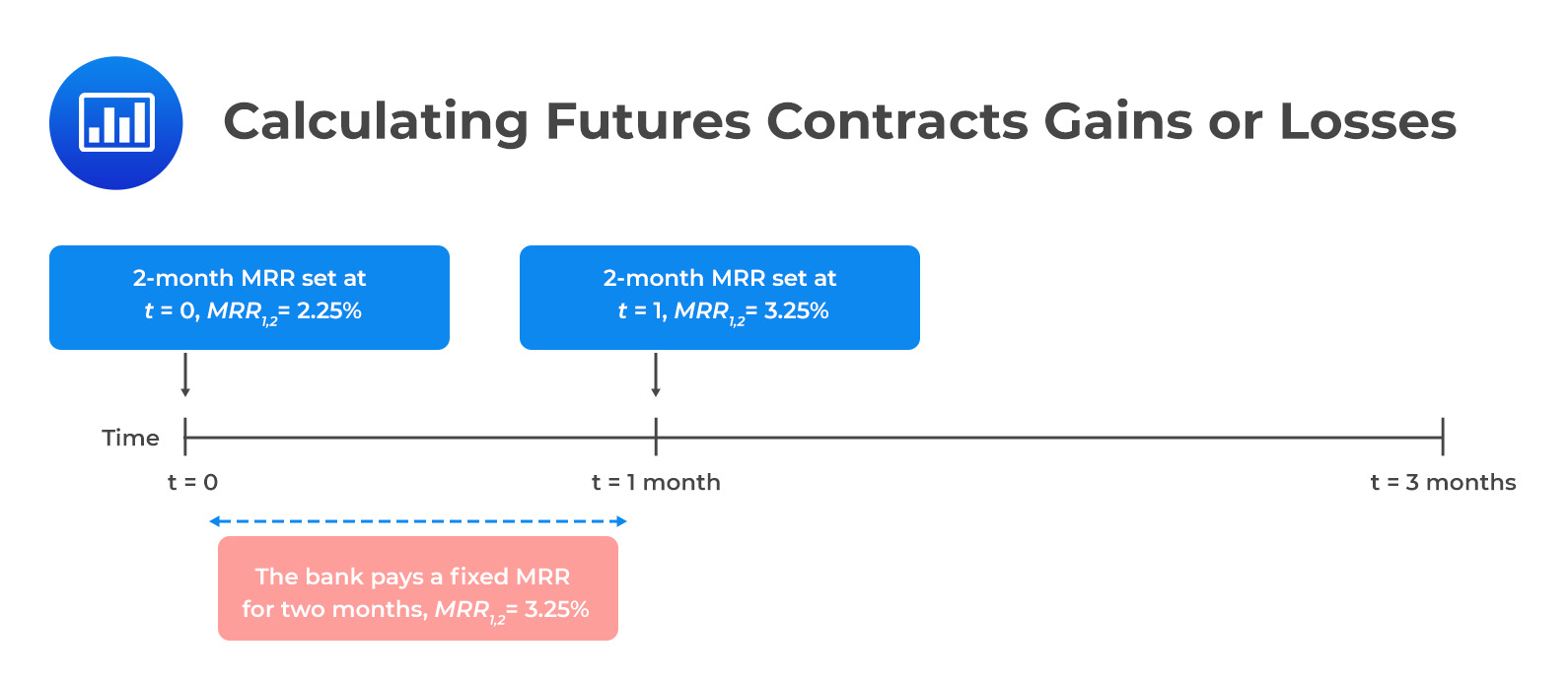# Value and Price of Futures Contracts

Recall that during the initiation of a forward commitment, no cash changes hands. Further, the forward commitment is neither a liability nor an asset to a buyer or the seller. As such, the value of both the forward contract and futures contract is zero:

$$V_0(T)=0$$

Consider an underlying with no associated costs or benefits. Like forward contracts, the futures price is calculated by compounding the spot price of the underlying using the risk-free rate:

$$f_T(0)=S_0(1+r)^T$$

Where:

$$f_T(0)=$$ Futures forward price.

$$S_0=$$ Spot price of the underlying at time $$t=0$$.

$$r=$$ Risk-free rate.

$$T=$$ Time to maturity.

Note that, like forward contracts, we have used discrete compounding. However, continuous compounding is also preferred in futures contracts if the underlying assets comprise a portfolio, such as commodities, fixed income, and equity. Also, continuous compounding is preferred when the underlying is foreign exchange denominated in two currencies.

Using continuous compounding, the future price is given by:

$$f_T(0)=S_0e^{rT}$$

## Cost of Carry and Futures Price

Like forward contracts, the price of futures whose underlying has income (I) and costs (C) is adjusted as follows:

$$f_T(0)=[S_0-PV_0(I)+PV_0(C)](1+r)^{T}$$

Where:

$$PV_0(I)=$$ Present value of income or benefit associated with the underlying at time $$t=0$$.

$$PV_0(C)=$$ Present value of costs associated with the underlying at time $$t=0$$

### Example: Futures Price Valuation

Minners Inc. enters a futures contract on an exchange via a financial intermediary to buy 80 kilos of gold. The current spot price is 52,950 per kilo. If the risk-free rate of return is 3%, what is the no-arbitrage futures price for settlement in 95 days? #### Solution The futures price is equal to the compounded value of the spot price of the underlying at the risk-free rate for a period $$T$$: \begin{align*}f_T(T)&=S_0(1+r)^T\\&=\52,950(1.03)^{\frac{95}{365}}\\&=\53,358.94\end{align*} ## Mark-to-Market Valuation of a Future Contract Compared to a Forward Contract As time passes, the value of futures and forward contracts changes. However, the forward contract price remains constant until maturity. As seen previously, for the long position, the value of a forward contract during its life is calculated as the difference between the current spot price and the present value of the original forward price: $$V_t(T)=S_t-F_0(T)(1+r)^{-(T-t)}$$ The MTM value of the forward contract is not settled until its expiration date, which causes counterparty risk. On the other hand, the futures price changes depending on market conditions. Moreover, the daily settlement resets the MTM value to zero. Besides, the variation margin is exchanged to cover the difference, decreasing counterparty risk. Note that the cumulative MTM gain or loss is approximately equal to that of a comparable forward contract. ## Interest Rate Futures and Forward Contracts Remember that a forward rate agreement (FRA) uses implied forward rates as a no-arbitrage fixed rate. In this instance, the counterparties exchange fixed for floating payments at a specified time in the future. The futures contracts on short-term interest rates are more liquid and standardized than FRAs. These contracts are often available for monthly and quarterly market reference rates (MRRs). ### Description of Interest Rate Futures As is the case in FRA, the underlying of the interest rate futures is the market reference rate on a hypothetical amount of money at a future date. However, interest rate futures trade on a price basis, given by the following formula: $$f_{A,B-A}=100-(100\times\text{MRR}_{A,B-A})$$ Where: $$f_{A,B-A}=$$ futures price for the market reference rate for $$B – A$$ periods that begin in A period $$(MRR_{A, B-A})$$.Note that the formula $$f_{A, B-A}=100-(100\times\text{MRR}_{A, B-A})$$ can be written as $$f_{A, B-A}=100-\text{yield}$$. Intuitively, the (100 – Yield) price convention leads to an inverse price versus yield relationship that differs from the price of a zero-coupon bond at a contract rate. As such, a long futures position receives MRR in A period while the short position pays MRR in A period. In summary, the long position (lender) gains as prices rise and future MRR falls. In contrast, the short position (borrower) gains as prices fall and future MRR rises. ### Interest Rate Futures Settlement The daily settlement of the interest futures occurs depending on the price changes, regarded as futures contract basis point value (BPV) and calculated as follows: $$\text{Futures contract BPV}=\text{Notional principlal}\times0.01\%\times\text{Period}$$ For instance, consider USD 50 million for a 3-month MRR of 3% for 180/360 days. The futures contract BPV is: $$\text{Futures contract BPV}=50,000,000\times0.01\%\times\bigg(\frac{180}{360}\bigg)=\2,500$$ #### Example: Calculating Futures Contracts Gains or Losses A&M Bank has issued its clients a USD 10 million three-month loan at a fixed rate. To finance the loan, the bank has borrowed a one-month variable MRR. To hedge against interest rate risk, the bank sells futures contracts on two-month MRR. Assume that the bank agrees to sell the futures at97.75, but the actual settlement price is \$96.75.

The cumulative gain/loss to the contract from the bank’s perspective is closest to:

#### Solution

We need to start by calculating the contract’s BPV:

\begin{align*}\text{Futures contract BPV}&=\text{Notional principal}\times0.01\%\times\text{Period}\\&=10,000,000\times0.01\%\times\frac{2}{12}\\&=\166.67\end{align*}

We need to calculate corresponding market reference rates (MRRs) for both prices.  Note that

$$f_{A, B-A}=100-(100\times\text{MRR}_{A, B-A})$$

Therefore;

\begin{align*}96.75&=100-(100\times\text{MRR}_{A, B-A})\rightarrow MRR_{1,2}=3.25\%\\ 97.75&= 100-(100\times\text{MRR}_{A, B-A})\rightarrow\text{MRR}_{1,2}=2.25\%\end{align*}

The bank has made a 100bps gain $$(=3.25\%-2.25\%)$$. In monetary terms, the bank has made a cumulative gain of $$\16,667 (= \text{Futures contract BPV} \times \text{100bps})$$ on the contract.## Question

Which of the following best describes the difference between the price of a futures contract and its value?

A. The price determines the profit to the buyer, and the value determines the profit to the seller.

B. The futures price is fixed at the start, and the value starts at zero and changes throughout the contract’s life.

C. The futures contract value is a benchmark against which the price is compared to determine whether a trade is advisable.

### Solution

The futures price is fixed at the start, whereas the value starts at zero and then changes, either positively or negatively, throughout the contract’s life.

Shop CFA® Exam Prep

Offered by AnalystPrepLevel I
Level II
Level III
All Three Levels
Featured Shop FRM® Exam PrepFRM Part I
FRM Part II
FRM Part I & Part II
Learn with Us

Subscribe to our newsletter and keep up with the latest and greatest tips for success
Shop Actuarial Exams PrepExam P (Probability)
Exam FM (Financial Mathematics)
Exams P & FM
Shop GMAT® Exam PrepComplete CourseSergio Torrico
2021-07-23
Excelente para el FRM 2 Escribo esta revisión en español para los hispanohablantes, soy de Bolivia, y utilicé AnalystPrep para dudas y consultas sobre mi preparación para el FRM nivel 2 (lo tomé una sola vez y aprobé muy bien), siempre tuve un soporte claro, directo y rápido, el material sale rápido cuando hay cambios en el temario de GARP, y los ejercicios y exámenes son muy útiles para practicar.diana
2021-07-17
So helpful. I have been using the videos to prepare for the CFA Level II exam. The videos signpost the reading contents, explain the concepts and provide additional context for specific concepts. The fun light-hearted analogies are also a welcome break to some very dry content. I usually watch the videos before going into more in-depth reading and they are a good way to avoid being overwhelmed by the sheer volume of content when you look at the readings.Kriti Dhawan
2021-07-16
A great curriculum provider. James sir explains the concept so well that rather than memorising it, you tend to intuitively understand and absorb them. Thank you ! Grateful I saw this at the right time for my CFA prep.nikhil kumar
2021-06-28
Very well explained and gives a great insight about topics in a very short time. Glad to have found Professor Forjan's lectures.Marwan
2021-06-22
Great support throughout the course by the team, did not feel neglectedBenjamin anonymous
2021-05-10
I loved using AnalystPrep for FRM. QBank is huge, videos are great. Would recommend to a friendDaniel Glyn
2021-03-24
I have finished my FRM1 thanks to AnalystPrep. And now using AnalystPrep for my FRM2 preparation. Professor Forjan is brilliant. He gives such good explanations and analogies. And more than anything makes learning fun. A big thank you to Analystprep and Professor Forjan. 5 stars all the way!michael walshe
2021-03-18
Professor James' videos are excellent for understanding the underlying theories behind financial engineering / financial analysis. The AnalystPrep videos were better than any of the others that I searched through on YouTube for providing a clear explanation of some concepts, such as Portfolio theory, CAPM, and Arbitrage Pricing theory. Watching these cleared up many of the unclarities I had in my head. Highly recommended.